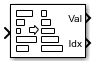# Sort

Sort input elements by value

• Library:
• DSP System Toolbox / Statistics

•## Description

The Sort block ranks the values of the input elements along each channel (column) in an `Ascending` or a `Descending` order, based on the Sort order you specify. Complex inputs are sorted by their magnitude, which is the sum of the squares of the real and imaginary components of the input. You can choose the Sort algorithm to be either ```Quick sort``` or `Insertion sort`. The quick sort algorithm uses a recursive sort method and is faster at sorting more than 32 elements. The insertion sort algorithm uses a nonrecursive method and is faster at sorting fewer than 32 elements. When you generate code, use the insertion sort algorithm to avoid recursive function calls.

The Mode parameter specifies the block's mode of operation, which you can set to `Value`, `Index`, or `Value and Index`.

## Ports

### Input

expand all

The block accepts real-valued or complex-valued multichannel inputs. The input data type must be double precision, single precision, integer, or fixed point, with power-of-two slope and zero bias.

Data Types: `single` | `double` | `int8` | `int16` | `int32` | `int64` | `uint8` | `uint16` | `uint32` | `uint64` | `fixed point`
Complex Number Support: Yes

### Output

expand all

The block sorts the data along each channel and outputs the sorted data through this port. The size, data type, and complexity of the sorted data matches that of the input data. The block sorts complex inputs according to their magnitude.

#### Dependencies

To enable this port, set the Mode parameter to `Value and index` or `Value`.

Data Types: `single` | `double` | `int8` | `int16` | `int32` | `int64` | `uint8` | `uint16` | `uint32` | `uint64` | `fixed point`
Complex Number Support: Yes

The output at this port contains the indices of the sorted data.

#### Dependencies

To enable this port, set the Mode parameter to `Value and index` or `Index`.

Data Types: `uint32`

## Parameters

expand all

### Main Tab

When the Mode parameter is set to:

• `Value` — The block sorts the elements in each channel of the M-by-N input matrix in an ascending or descending order, based on what you specify in the Sort order parameter. The output at each sample time, Val, is an M-by-N matrix that contains the sorted columns of the input.

The block sorts complex inputs according to their magnitude.

• `Index` — The block sorts the elements in each channel of the M-by-N input matrix, and outputs the index array, I. Each element in I is an integer of type `uint32` that indexes the sorted value in the corresponding column of the input.

• `Value and index` — The block outputs the sorted values of the input data, Val, and the corresponding indices in the index array, I.

Specify to sort the input data in either ascending or descending order.

The quick sort algorithm uses a recursive sort method and is faster at sorting more than 32 elements. The insertion sort algorithm uses a nonrecursive method and is faster at sorting fewer than 32 elements. When you generate code, to avoid recursive function calls, use the insertion sort algorithm.

### Data Types Tab

Note

To use these parameters, the data input must be complex and fixedpoint. For all other inputs, the parameters on the Data Types tab are ignored.

Specify the rounding mode for fixed-point operations. For more details, see rounding mode.

When you select this parameter, the block saturates the result of its fixed-point operation. When you clear this parameter, the block wraps the result of its fixed-point operation. For details on `saturate` and `wrap`, see overflow mode for fixed-point operations.

The squares of the real and imaginary parts of the complex input are stored in the Product output data type.

You can set this parameter to:

• `Inherit: Same as input` — The product output data type is the same as the input data type.

• `fixdt([],16,0)` — The product output data type is an autosigned, binary-point, scaled, fixed-point data type with a word length of 16 bits and a fraction length of 0.

Alternatively, you can set the Product output data type by using the Data Type Assistant. To use the assistant, click the button.

For more information on the data type assistant, see Specify Data Types Using Data Type Assistant (Simulink).

The result of the sum of the squares of the real and imaginary parts of the complex input are stored in the Accumulator data type.

You can set this parameter to:

• `Inherit: Same as product output` — The accumulator data type is the same as the product output data type.

• `Inherit: Same as input` — The accumulator data type is the same as the input data type.

• `fixdt([],16,0)` — The accumulator data type is an autosigned, binary-point, scaled, fixed-point data type with a word length of 16 bits and a fraction length of 0.

Alternatively, you can set the Accumulator data type by using the Data Type Assistant. To use the assistant, click the button.

For more information on the data type assistant, see Specify Data Types Using Data Type Assistant (Simulink).

Select this parameter to prevent the fixed-point tools from overriding the data types you specify on the block.

## Block Characteristics

 Data Types `double` | `fixed point` | `integer` | `single` Direct Feedthrough `no` Multidimensional Signals `no` Variable-Size Signals `no` Zero-Crossing Detection `no`

expand all

## Version History

Introduced before R2006a